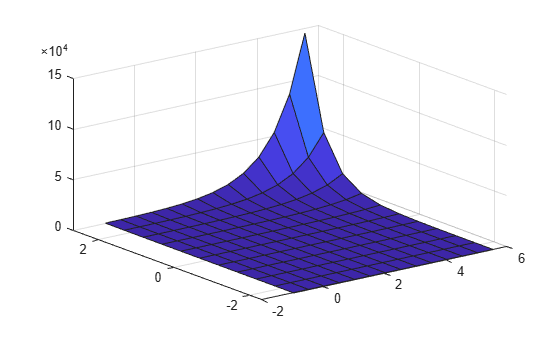# feval

Predict responses of generalized linear regression model using one input for each predictor

## Syntax

``ypred = feval(mdl,Xnew1,Xnew2,...,Xnewn)``

## Description

example

````ypred = feval(mdl,Xnew1,Xnew2,...,Xnewn)` returns the predicted response of `mdl` to the new input predictors `Xnew1,Xnew2,...,Xnewn`.```

## Examples

collapse all

Create a generalized linear regression model, and plot its responses to a range of input data.

Generate sample data using Poisson random numbers with two underlying predictors `X(:,1)` and `X(:,2)`.

```rng('default') % For reproducibility rndvars = randn(100,2); X = [2 + rndvars(:,1),rndvars(:,2)]; mu = exp(1 + X*[1;2]); y = poissrnd(mu);```

Create a generalized linear regression model of Poisson data.

`mdl = fitglm(X,y,'y ~ x1 + x2','Distribution','poisson');`

Generate a range of values for `X(:,1)` and `X(:,2)`, and plot the predictions at the values.

```[Xtest1,Xtest2] = meshgrid(min(X(:,1)):.5:max(X(:,1)),min(X(:,2)):.5:max(X(:,2))); Z = feval(mdl,Xtest1,Xtest2); surf(Xtest1,Xtest2,Z)```## Input Arguments

collapse all

Generalized linear regression model, specified as a `GeneralizedLinearModel` object created using `fitglm` or `stepwiseglm`, or a `CompactGeneralizedLinearModel` object created using `compact`.

New predictor values, specified as a vector, matrix, table, or dataset array.

• If you pass multiple inputs `Xnew1,Xnew2,...,Xnewn` and each includes observations for one predictor variable, then each input must be a vector. Each vector must have the same size. If you specify a predictor variable as a scalar, then `feval` expands the scalar argument into a constant vector of the same size as the other arguments.

• If you pass a single input `Xnew1`, then `Xnew1` must be a table, dataset array, or matrix.

• If `Xnew1` is a table or dataset array, it must contain predictors that have the same predictor names as in the `PredictorNames` property of `mdl`.

• If `Xnew1` is a matrix, it must have the same number of variables (columns) in the same order as the predictor input used to create `mdl`. Note that `Xnew1` must also contain any predictor variables that are not used as predictors in the fitted model. Also, all variables used in creating `mdl` must be numeric. To treat numerical predictors as categorical, identify the predictors using the `'CategoricalVars'` name-value pair argument when you create `mdl`.

Data Types: `single` | `double` | `table`

## Output Arguments

collapse all

Predicted response values at `Xnew1,Xnew2,...,Xnewn`, returned as a numeric vector.

For a binomial model, `feval` uses 1 as the `BinomialSize` parameter, so the values in `ypred` are predicted probabilities. To return the numbers of successes in the trials, use the `predict` function and specify the number of trials by using the `'BinomialSize'` name-value pair argument.

For a model with an offset, `feval` uses 0 as the offset value. To specify the offset value used when you fit a model, use the `predict` function and the `'Offset'` name-value pair argument.

## Tips

• A regression object is, mathematically, a function that estimates the relationship between the response and predictors. The `feval` function enables an object to behave like a function in MATLAB®. You can pass `feval` to another function that accepts a function input, such as `fminsearch` and `integral`.

• `feval` can be simpler to use with a model created from a table or dataset array. When you have new predictor data, you can pass it to `feval` without creating a table or matrix.

## Alternative Functionality

• `predict` gives the same predictions as `feval` if you use the default values for the `'Offset'` and `'BinomialSize'` name-value pair arguments of `predict`. The prediction values can be different if you specify other values for these arguments. The `predict` function also returns confidence intervals on its predictions. Note that the `predict` function accepts a single input argument containing all predictor variables, rather than multiple input arguments with one input for each predictor variable.

• `random` predicts responses with added noise.

## Version History

Introduced in R2012a